# 2034: Equations

 EquationsTitle text: All electromagnetic equations: The same as all fluid dynamics equations, but with the 8 and 23 replaced with the permittivity and permeability of free space, respectively.

## Explanation

This comic gives a set of mock equations. To anyone not familiar with the field in question they look pretty similar to what you might find in research papers or on the relevant Wikipedia pages. Most of the jokes are related to the symbols or "look" of most equations in the given field.

The comic makes jokes about the fields of kinematics, number theory, fluid dynamics, quantum mechanics, chemistry, quantum gravity, gauge theory, cosmology, and physics equations. Of course, all of the equations listed are not real equations (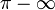$\pi-\infty$ and H2EAT are clearly jokes and making a mockery of the given field). As always, Randall is just having a laugh.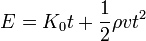$E=K_{0}t+\frac{1}{2}\rho{}vt^2$
All kinematics equations

Most kinematics equations tend to make heavy use of constants, addition, powers, and multiplication. This specific equation resembles the actual kinematics equation d = vt + 1/2at^2, but replaces a (acceleration) with v (velocity) times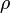$\rho{}$ (density) and replaces velocity with "K0", which is not a term used in kinematics.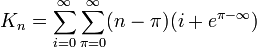$K_{n}=\sum_{i=0}^{\infty}\sum_{\pi=0}^{\infty}(n-\pi)(i+e^{\pi-\infty})$
All number theory equations

Randall jokes about how number theory often involves the use of summations. The use of π as an integer variable in the double summation is a joke, as π is essentially always used for the well-known constant 3.14159..., not a variable. The use of i as a summation variable is common, though it can also be confused with the imaginary unit √-1. The constants e, i, and π, as well as the theoretical upper bound$\infty$, often appear in number theory equations.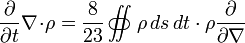$\frac{\partial}{\partial{t}}\nabla\!\cdot\!\rho=\frac{8}{23}\int\!\!\!\!\int\!\!\!\!\!\!\!\!\!\subset\!\!\supset\rho\,{ds}\,{dt}\cdot{}\rho\frac{\partial}{\partial\nabla}$
All fluid dynamics equations

Fluid dynamics equations often involve copious integrals, especially those over closed contours as done here, which are often the main telling factors of those equations to an outsider. The time derivative and gradient operator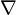$\nabla$ are common in fluid dynamics, mostly via the Navier-Stokes equation, and the fluid density$\rho$ is one of the functions of central importance. The fraction 8/23 is a comically weird choice, but various unexpected fractions do pop up in fluid dynamics. The ds and dt go with the double contour integral (s is probably distance, t is time), but the derivative with respect to$\nabla$ at the end is very much not allowed.$|\psi_{x,y}\rangle=A(\psi)A(|x\rangle\otimes|y\rangle)$
All quantum mechanics equations

Quantum mechanics often involves some of the foreign-looking symbols listed, including bra-ket notation, the tensor product, and the Greek letter Psi for a quantum state. Specifically, the left side of the equation is a ket state labeled Psi that depends on x and y (probably positions), while the right-hand side may be an operator A that depends on the state Psi (it is very unusual to have such a dependence) acting on what looks like another copy of that operator which depends on the outer product of states labeled by x and y (again strange). A charitable interpretation could be that the second A is the eigenfunction A of the operator A. Normally this is clearly indicated by giving the operator a “hat” (^ symbol) or making the eigenfunction into a ket eigenstate, but since the equation is intentionally nonsense both A’s are left ambiguous. Also note that the bra-ket math is inconsistent here, as the left side is a ket, but the right side is just two A’s, which are either operators or functions but are definitely not kets.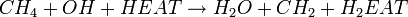$CH_4+OH+HEAT\rightarrow{}H_2O+CH_{2}+H_2EAT$
All chemistry equations

Chemistry equations use formulas of chemical compounds to describe a chemical reaction. Such equations show the starting chemicals on the left side and the resulting products on the right side, as displayed. Sometimes such an equation might optionally indicate that an activation energy is required, for the reaction to take place in a sensible timeframe, e.g. by heating. A reaction requiring heating is usually indicated by a Greek capital letter Delta (Δ) or a specified temperature above the reaction arrow, however this comic uses the "+ HEAT" term on the left side instead. The joke is that Randall interprets "HEAT" to be another chemical, which reacts with Hydrogen (H) to H2EAT, which is nonsensical, as heat is transferred energy here, not added matter. Regardless of this, Randall gets the stoichiometry of this equation correct, with the same number of all types of 'atoms' on each side of the equation.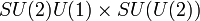$SU(2)U(1)\times{}SU(U(2))$
All quantum gravity equations

Quantum gravity uses mathematical groups denoted by uppercase letters, as shown. SU(2), U(1), and U(2) are all well-studied groups, though 'SU(U(2))' makes no sense. The lack of relator means this expression isn't an equation. Here is a possible pun, on "Sue you too... you won"... "Sue you, you too", though it's unclear how it fits in here.

Sg=(-1)/(2ε̄) ið(̂ ξ0 ⨢ pε ρvabc⋅η0)̂ f̵a0 λ(ξ) ψ(0a)
All gauge theory equations

Gauge theory is a subset of field theory. Most gauge theory equations appear to have many strange-looking constants and variables with odd labels.$H(t)+\Omega+G\!\cdot\!\Lambda...\begin{cases}...>0\mathsf{\ (Hubble\ model)}\\ ...=0\mathsf{\ (Flat\ sphere\ model)}\\ ...<0\mathsf{\ (Bright\ dark\ matter\ model)} \end{cases}$
All cosmology equations

Cosmology is the science of the development and ultimate fate of the universe. The joke here may be pertaining to the different models accepted in the field of cosmology. H is the Hubble parameter, Ω is the universal density parameter, G is the gravitational constant, and Λ is the cosmological constant.

Ĥ - u̧0 = 0
All truly deep physics equations

The joke about the "truly deep physics equations" is that most of the universal physics equations are simple, almost exceedingly so. One example is Einstein's E = mc².

The title text is referencing the fact that the electric and magnetic fields are often explained to physics students using an analogy with fluid dynamics, as well as the fact that they do share some similarities (only in terms of mathematical description as three-dimensional vector fields) with fluids. The permittivity constant (represented with ε0) and the permeability constant (represented with μ0) are coefficients that relate the amount of charge required to cause a specific amount of electric flux in a vacuum and the ability of vacuum to support the formation of magnetic fields, respectively. They appear frequently in Maxwell's equations (the equations that define the electric and magnetic fields in classical mechanics), so Randall is making the joke that any surface integral with them in it automatically is an electromagnetism equation.

## Transcript

[Nine equations are listed, three in the top row and two in each of the next three rows. Below each equation there are labels:]
E=K0t+1/2 ρvt2
All kinematics equations
Kn=∑i=0π=0(n-π)(i-eπ-∞) [K sub n = the summation from i = 0 to infinity of the sum from pi = 0 to infinity of (n - pi) * (i-e^(pi-infinity))]
All number theory equations
∂/∂t ∇⋅ρ=8/23 (∯ ρ ds dt ⋅ ρ ∂/∂∇)
All fluid dynamics equations
x,y〉=A(ψ)A(|x〉⊗|y〉)
All quantum mechanics equations
CH4+OH+HEAT→H2O+CH2+H2EAT
All chemistry equations
SU(2)U(1)×SU(U(2))
All quantum gravity equations
Sg=(-1)/(2ε̄) ið(̂ ξ0 ⨢ pε ρvabc⋅η0)̂ f̵a0 λ(ξ) ψ(0a)
All gauge theory equations
H(t)+Ω+G⋅Λ ...
[There is a brace linking the three cases together.]
... > 0 (Hubble model)
... = 0 (Flat sphere model)
... < 0 (Bright dark matter model)
All cosmology equations
Ĥ - u̧0 = 0
All truly deep physics equationsadd a comment! ⋅add a topic (use sparingly)! ⋅refresh comments!

# Discussion

Is the joke that all of the equations are actually wrong/malformed/meaningless but they sort of look like typical equations for that field? 172.68.133.66 (talk) (please sign your comments with ~~~~)

Sort of. A bit of dimensional analysis would have helped. ;-) --162.158.91.221 07:28, 17 August 2018 (UTC)
I feel that the joke is that all the equations are very complicated, and use multiple letters and symbols. But the last one "Truly deep Physics Equations" are summed up with just 3 characters and 2 basic operators. 108.162.250.11 10:56, 19 August 2018 (UTC)

He's nerd sniping us all.. (162.158.167.120 03:30, 18 August 2018 (UTC))

..and perhaps forcing us to build out symbolic usage which is not generating well for math parsers, wiki, etc. 162.158.186.60 14:45, 21 August 2018 (UTC)

Should we add a column with examples of similar correct equations from the respective fields? Sebastian --172.68.110.4 09:33, 17 August 2018 (UTC)

That would definitely tidy up my attempts to provide context for Randall's versions. The challenge then is working in explanations for the correct equations as well as arguing over which examples should be used. Exxi (talk) 09:45, 17 August 2018 (UTC)

I don't think the part in parentheses about OH in the Chemistry equation explanation is correct. OH- would mean that it's negatively charged and has nothing to do with unpaired electrons of Oxygen. It would add another horror to the equation, though, as it wouldn't be charge preserving anymore. 162.158.88.230 09:58, 17 August 2018 (UTC)

"Redshit". Best typo ever. Please keep it. 172.69.54.177 10:13, 17 August 2018 (UTC)

Deep physics equations

The transcript is wrong here, the last letter is not a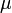$\mu$, but a "u" with a cedilla: u̧. The math parser refuses to render it, though. 162.158.88.230 05:54, 17 August 2018 (UTC)

Looks like it. But I don't think that letter exists even. --162.158.91.221 07:28, 17 August 2018 (UTC)
Is this equation a sort of nod to a Theory Of Everything which unifies quantum mechanics and gravity... H-hat (a Hamiltonian, which in quantum mechanics describes the total energy of a system, and usually runs in to problems describing large systems - such as the entire universe - where gravity or spacetime curvature effects matter) *minus* u0 (the relativistic mass of the whole system at time zero ie. the big bang) gives 0 (no energy everywhere always). Since mass is energy (e=mc^2) and mass is also the sole cause of gravity the two theories cleanly collapse together when mass is zero, and figuring out how to extend the theory to other less clean points on the mass axis is obviously a job for less profound physics? I've no ideas to explain the cedilla. 141.101.98.28 08:49, 17 August 2018 (UTC)
E=mc^2 no more means that vis is mass than V=abc means that volume is width; rather they are proportional. Lysdexia (talk) 01:42, 5 January 2019 (UTC)
It looks to me a little like a parody of the Wheeler-DeWitt equation which (in theory) describes a wavefunction for the entire Universe. Exxi (talk) 09:06, 17 August 2018 (UTC)一
I'm just thrilled someone found the right character for it. I spent 20 minutes looking for the right u symbol without any luck at all. 172.68.143.132 (talk) (please sign your comments with ~~~~)

Is this poking fun at equation-filled blackboards in movies and cartoons? 172.68.254.42 (talk) (please sign your comments with ~~~~)

Doesn't seem like it. These equations actually do look like the kinds of equations you would see in these fields. On blackboards in movies you tend to get equations that are pure nonsense. 172.68.143.132 (talk) (please sign your comments with ~~~~)
pure = clean -> sheer = absolute Lysdexia (talk) 01:42, 5 January 2019 (UTC)

I think this may also be a reference to Feynman's unworldliness equation, http://www.feynmanlectures.caltech.edu/II_25.html#Ch25-S6 . 108.162.219.220 17:02, 17 August 2018 (UTC)

I'd thought the point of the "u" with the cedilla was simply visual (not a reference to an actual parameter). It visually looks like the head of "The Thinker" in profile with the bottom of the "u" being the chin and the cedilla being the curled hand upon which the chin rests. So, the joke would be a twist on the term "deep" where in the cartoon the u+cedilla represents deep thought rather than far away (deep) into space. Monroe likes these little visual puns.Genejockey33000 (talk) 14:01, 22 August 2018 (UTC)

I don't like the comparison with$E=mc^2$. 'Deep' physics equations have a lot of meaning assigned to each symbol, with each prime, tilde or cedilla adding meaning, and with complicated operations implicit (Think operators, tensor products etc.). Einstein's relation is just a relation between 3 scalar variables, with the only operation being multiplication. I appreciate not wanting to get too complicated. Can we do any better? I can't help thinking that writing down the GFEs in natural units and saying 'this describes all of spacetime' owtte would be just as effective and more accurate...Jezza2 (talk) 16:03, 24 January 2019 (UTC)

Table layout at the explanation

That oversized table is really bad layout. We've had this discussion many times before - tables should only be used for small contents. Right now I would run into too many edit conflicts but I'll change it to a proper floating text with small headers for each section. --Dgbrt (talk) 11:51, 17 August 2018 (UTC)

Done, looks much more like a real paper... --Dgbrt (talk) 12:58, 17 August 2018 (UTC)
All number theory equation

The explanation for math doesn't seem entirely correct. You can in fact extend the ring of integers (as well as rational and real numbers) with positive and negative infinity, but it won't be a ring anymore. Specifically, the infinities don't have an additive or multiplicative inverse (but 1/infinity = 0); and addition of positive and negative infinity, as well as the product of 0 and either infinity is undefined. However, these properties are not used in the above equation. What we can use is that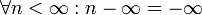$\forall n < \infty: n - \infty= -\infty$. We would thus have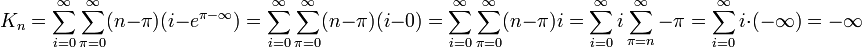$K_n = \sum_{i=0}^{\infty}\sum_{\pi=0}^{\infty}(n-\pi)(i-e^{\pi-\infty}) = \sum_{i=0}^{\infty}\sum_{\pi=0}^{\infty}(n-\pi)(i-0) = \sum_{i=0}^{\infty}\sum_{\pi=0}^{\infty}(n-\pi)i= \sum_{i=0}^{\infty}i\sum_{\pi=n}^{\infty}-\pi= \sum_{i=0}^{\infty}i\cdot(-\infty)=-\infty$. Also, how often does one use e and pi in number theory? --Ycthiognass (talk) 12:11, 17 August 2018 (UTC)

Pi (or any other number) minus infinite is just absurd. You can use the infinite symbol only as a limit but NOT as number in calculations. --Dgbrt (talk) 12:33, 17 August 2018 (UTC)
It is not absurd. Adding the rules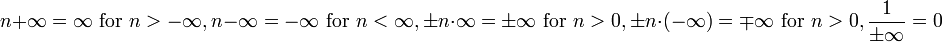$n+\infty=\infty\text{ for }n>-\infty,n-\infty=-\infty\text{ for }n<\infty, \pm n\cdot\infty = \pm\infty\text{ for }n>0, \pm n\cdot(-\infty) = \mp\infty\text{ for }n>0,\frac1{\pm\infty}=0$ gives you a consistent theory that is especially useful when talking about infinite sums and integrals. Would you say the term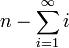$n-\sum_{i=1}^\infty i$ is absurd? --Ycthiognass (talk) 14:35, 17 August 2018 (UTC)
Of course it's absurd. It is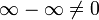$\infty-\infty \neq 0$ because it could be everything between$\infty$ and$-\infty$. Infinity is a concept describing something without any bound... And, as you can't divide by zero you can't do the same for infinity. --Dgbrt (talk) 17:24, 17 August 2018 (UTC)
One more: It is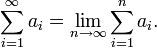$\sum_{i=1}^\infty a_i = \lim_{n\to\infty} \sum_{i=1}^n a_i.$
When this limit exists, one says that the series is convergent or summable. Otherwise it's called divergent and has no solution like this one:$\sum_{i=1}^\infty i$
Infinite is NO number! --Dgbrt (talk) 17:33, 17 August 2018 (UTC)$\infty-\infty$ is indeterminate, just like 0/0 is in standard arithmetic. That's cool, because we don't need the value of$\infty-\infty$ to calculate the above expression. Have a look at the extended real number line. --Ycthiognass (talk) 06:18, 20 August 2018 (UTC)
1/0 != ∞ and 1/∞ != 0 wherefore 1 != 0∞ wherefore 0∞ = ℝ, and -∞ != -1 != 0 != 1 != 2 != ∞; therefore 1/0 ⊂ ∞, 1/∞ ⊂ 0, 1/0 ⊂ ±∞, and 1/±∞ ⊂ 0. Lysdexia (talk) 01:42, 5 January 2019 (UTC)
Number theorists use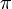$\pi$ and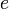$e$ very often indeed, especially analytic number theorists (and since the double sum at hand is infinite it seems entirely fair to consider it analytic number theory territory). The exponential function$e(x) = e^{2 \pi i x}$ is used all over in this field because of Fourier series (the coefficients of which, it turns out, can give remarkable amounts of arithmetic information if used properly).
The same field of analytic number theory uses the infinity symbol$\infty$ in calculations quite frequently too, because it is not uncommon to work on the Riemann sphere, which is the complex plane with a "point at infinity added". (That said, it doesn't quite save our hides in this particular comic, because the point at infinity doesn't have an additive inverse in this context.)
For the record there are also numerous ways to extend the real numbers to include infinities in more or less algebraically nice ways, ranging from compactifying it by just tacking a point at infinity on (as with the Riemann sphere for the complex numbers, also called a projective extension), tacking a positive and negative infinity on as with the extended real line as above, to more algebraically nice structures like the hyperreals and surreals which are full blown fields. In these latter constructions it is not customary to use the$\infty$ symbol, however, because there is more than just the one infinity in both, but they do allow us to make meaningful and useful sense of things like "infinity - infinity". Prstq (talk)
Don't make nice < niais < nescius := not-skilled ways and structures. ∞ := ℵ₀. Lysdexia (talk) 01:42, 5 January 2019 (UTC)

Completely separate from the above, it's probably worth noting that i is also a constant, and as such has the same misconception as$\pi$. Computer scientists are happy using i for loops/summations, but mathematicians prefer using n. Based off that, it's probably another misconception/joke that n is treated as a constant, while known-constants are used as variables. 108.162.246.149 17:28, 17 August 2018 (UTC)

There is nothing non-standard about using i as an index variable. Often as part of the series i,j,k. Searching for summation convention will give plenty of examples.
There are fewer letters than mathematical concepts in need of letters, so most letters are used for multiple purposes. Occasionally this causes difficulty. You can be halfway through a linear algebra problem before you discover you need i for an imaginary number despite already using it as an index. Hilarity ensues. 162.158.74.105 19:57, 17 August 2018 (UTC)

Is it worth mentioning Euler's identity in the explanation? As a non-mathematician, the presence of e, pi, and i together in one equation looks "Euler's identity-ish" while clearly not being it. 162.158.74.39 22:20, 19 August 2018 (UTC)

Honestly, seeing e, pi, and i isn't that farfetched, since quite a few applications of number theory to coordinate systems (in the computer-ey sense) take familiar XY coordinates, make them polar, and call them to complex numbers, with a bunch of operations done by multiplying by e to some complex power or other. And wherever waves go, pi goes... Coming from nothing more than a high-school background, this whole system can be rather jarring, and difficult to understand. I'm just glad I understand enough bits and pieces of number theory to laugh at Randall's joke. 162.158.186.12 19:41, 1 September 2018 (UTC)

Chemistry equation

OH should have a charge symbol: OH-. The actual reaction would be:

CH4 + OH- + heat -> CH3- + H2O

The methyl group can dissolve in water, and this is presumably happening in water, so this equation can work, just not the one provided by Randell. Reacting longer alkanes with bases is a way to make soaps, but the methyl group would be too reactive to be used this way. Nutster (talk) 13:13, 17 August 2018 (UTC) OH without any charge is the hydroxil radical, a highly reactive moleculte, that in fact abstracts H (hydrogen atom, not a proton) from hydrocarbons. This is one step of combustion processes. The methyl radical generated combines itself quickly with oxygen. The hydroxide anion you propose OH- do not react with hydrocarbons.

Fluid Dynamics equation

I believe the fraction 8/23 in the Fluid Dynamics equation is a Randallesque reference to the fractional approximation of pi = 22/7. It's probably not a coincidence that you get 8/23 from 22/7 if you invert it and add 1 to both the numerator and denominator. Ianrbibtitlht (talk) 15:19, 17 August 2018 (UTC)

I think that is a bit of a stretch. If Randall wanted to reference the 22/7 approximation, I think he would simply use 22/7 and not 8/23. Redbelly98 (talk) 00:40, 18 August 2018 (UTC)
It might be a stretch, but maybe Randall wanted to be more clever than just inserting 22/7, since pi really has no place in that equation. I don't see anyone else suggesting any reasonable source for 8/23 in the equation. The current explanation is an even bigger stretch, since it has nothing in common with 8/23 beyond being just another fraction - it seems to suggest he picked two random numbers, 8 and 23, for the fraction! How unsatisfying! But if nobody else agrees, I'm not losing any sleep over it. (Sometimes I wish Randall would chime in to clear things like this up for us. Randall, where are you?) Ianrbibtitlht (talk) 04:54, 18 August 2018 (UTC)
Fluid dynamicist here -- strange looking numbers and fractions come from multiplying tensors. 2/3 is a common one, but you also get numbers like 1/7 and 8/27.108.162.241.166 07:24, 18 August 2018 (UTC)

I changed a "p" to a Greek "rho". Redbelly98 (talk) 00:40, 18 August 2018 (UTC)

The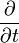$\frac{\partial}{\partial t}$ is the partial derivative of a function. The ∇ symbol (Nabla) denotes the vector of all partial derivatives of a function (called its gradient; eg. ∇(x+2y²)=(1,4y) ); ∇⋅ is the curl operator. 162.158.91.59 18:53, 5 December 2018 (UTC)

Gauge theory equation

I think the transcript is missing a left superscript 0 before the turned xi. 172.68.226.16 16:50, 17 August 2018 (UTC) Ah no, sorry. False alarm. It's just that Randall writes the xi with a funny tail. The same tail is on the non-turned xi earlier. 172.68.226.10 16:52, 17 August 2018 (UTC)

If explainxkcd.com is to make XKCD comics more understandable then this explanation is failing that. I assumed from the beginning that the joke was about the equations being wrong, but the description of the joke is making my head hurt. 162.158.106.216 (talk) (please sign your comments with ~~~~)

Read the first paragraph: "To someone who knows even a little about the topic, they are clearly very wrong and only seem even worse the more you look at them." Nevertheless the rest sometimes does hurt. See below. --Dgbrt (talk) 20:28, 17 August 2018 (UTC)
All truly deep physics equations

In the description paragraph, the last sentence starting "The principle of least action says allows..." does not scan. If someone can fix this (copy&paste?) error, please delete this comment. 162.158.58.171 19:33, 17 August 2018 (UTC)

I edited the sentence slightly to address this issue. Ianrbibtitlht (talk) 20:51, 17 August 2018 (UTC)

It looks like there is already a section for this above, "Deep physics equations", or am I missing something? Redbelly98 (talk) 00:40, 18 August 2018 (UTC)

Note that this comics also emphasizes that Randall is more familiar with physics than with chemistry : while most of the equations here require college-level education to grok, the chemistry one is at the very most high-scool-grade. 141.101.69.33 (talk) (please sign your comments with ~~~~)

Could this be a reference to Feynman's jab at hiding complexity underneath symbol definitions to achieve 'simplicity'? See the Feynman Lectures on Physics, Volume II, Chapter 25, Section 6. 162.158.150.100 09:19, 19 August 2018 (UTC)WhoIsJack

Sounds good to me. Exxi (talk) 19:29, 23 August 2018 (UTC)
Explanations in general

“Nobody knows if Randall references a horse here” - what?! Because the expression lacks an equal sign; doesn’t represent an equality, it might mean Randall is referencing equines, aka horses?! Is this vandalism, an attempt at a joke, or what? This explanation clearly still needs quite a bit of work! PotatoGod (talk) 20:14, 17 August 2018 (UTC)

I've put a header on top here. It's not vandalism but every explanation looks still highly unscientific. I've gave real sources to the most topics at the beginning but the following explanations are mostly bad. --Dgbrt (talk) 20:22, 17 August 2018 (UTC)
I left that joke in as it was added whilst I was editing the rest of it, I don't think it belongs though. I did my best to reference real-life stuff while writing [Gauge, Quantum Gravity and Cosmology] the awfulness of the equations makes it hard to be scientific though. If there are specific issues I can have a shot at improving those sections although it's kinda hard to explain why I find them funny without going deep into the related physics. I'm not convinced it's possible to properly get that across to a non-physicist in a paragraph of explainXKCD. Exxi (talk) 19:29, 23 August 2018 (UTC)
Kinematics equations

I fixed an error: Randall's Greek "rho" ρ, a common symbol for mass density, was incorrectly shown here as p, the common symbol for momentum. The term with the ρ is very similar to a term in the Bernoulli equation, and I have changed the explanation to reflect this. Redbelly98 (talk) 00:40, 18 August 2018 (UTC)

You might be overthinking some of these

For example, I see the first one as Energy = cot + private which would be an Army private resting in a cot to regain their energy. The second one I see the word Knee, so I'm thinking it's either something about taking an arrow to a knee, or perhaps about the Knights of Ki who regain their power by saying "Ni!" The fourth one, I see most of the word ANALOGY, so perhaps the trident-shaped thing equals N, and x> = L, and l (or 1) = G, so if you resolve all the way through you get GNL = ANALOGY and I don't have that quite right yet. SU(2)U(1)xSU(U(2)) makes me think of Phil Collins singing "Su-Su-Sussudio oh oh". --108.162.245.40 20:37, 18 August 2018 (UTC)

Has anyone considered the joke part?

As someone unfamiliar with scientific equations, I took the joke to be that Scientific Equations Are Complicated, until you get to the "truly deep" part, in which case they're pretty simple. As much as I appreciate the description of the equations, is anyone gonna explain whether my take on the joke is plausible? Or what it is if I'm wrong? 108.162.219.214 15:52, 20 August 2018 (UTC)

Is there a pun?

Am I the only one seeing a possible pun in SU(2)U(1)xSU(U(2))? I can't figure out the whole thing but SU(U(2)) sure looks like it reads "sue you too". 24.165.207.66 23:02, 20 August 2018 (UTC)

No element symbols exist to form EAT

Although you could write HeAt (Helium-Astatine), I could find no element symbols to form EAT on the right-hand side of the reaction, so it appears to be an invalid expression anyway. (and yes, of course I know Randall is messing with us and you might be expected to read the first "heat" as "temperarature".) --IByte (talk) 11:17, 4 September 2018 (UTC)

And Randall wrote HEAT, not HeAt. They are different. Redbelly98 (talk) 18:33, 9 September 2018 (UTC)
Heat (Q) is not interchangeabil with temperature (T). EAT could mean element-acid group-tritium or obsoletely erbium-argon-tritium. Lysdexia (talk) 01:42, 5 January 2019 (UTC)

Why does the notice still say 2034 lacks a complete explanation when here's no incomplete template? Lysdexia (talk) 01:42, 5 January 2019 (UTC)

Missing alt-text 141.101.77.104 12:19, 19 January 2019 (UTC)